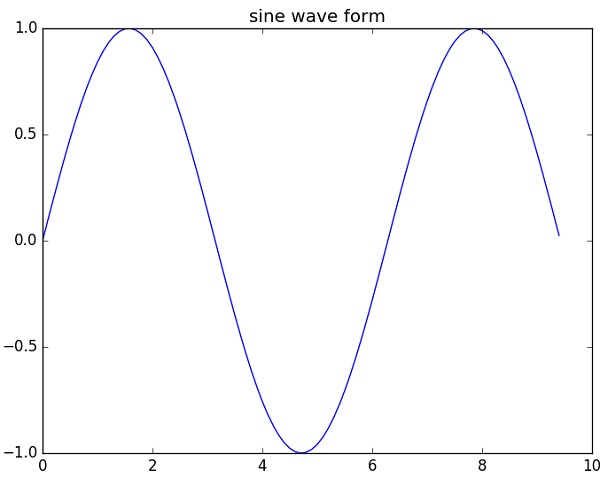# (7)Python Matplotlib库

Matplotlib是一个Python库，用于通过使用python脚本创建二维图形和图表。 它有一个名为pyplot的模块，通过提供控制线条样式，字体属性，格式化轴等功能，使得绘图变得容易。它支持各种各样的图形和图形 – 即直方图，条形图，功率谱，误差图等 它与NumPy一起使用，为MatLab提供了一个有效的开源替代方案。 它也可以用于像PyQt和wxPython这样的图形工具包。

``````from matplotlib import pyplot as plt
```Shell```

## Matplotlib示例

``````import numpy as np
import matplotlib.pyplot as plt

# Compute the x and y coordinates for points on a sine curve
x = np.arange(0, 3 * np.pi, 0.1)
y = np.sin(x)
plt.title("sine wave form")

# Plot the points using matplotlib
plt.plot(x, y)
plt.show()
```Shell```